Next: The Fixed Points of Up: Linear Algebra and Homogeneous Previous: Linear Algebra and Homogeneous

## Lines in the Plane and Incidence

We will develop the theory of lines in the projective plane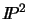. Most of the results can also be extended to higher dimensions.

Let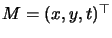and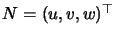be homogeneous representatives for two distinct points in the plane (i.e. M and N are linearly independent 3-vectors: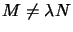). Let L=(a,b,c) be a the dual coordinates of a line (hyperplane) in the plane. By the definition of a hyperplane, M lies on L if and only if the dot product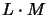vanishes, i.e. if and only if the 3-vector L is orthogonal to the 3-vector M. The line MN through M and N must be represented by a 3-vector L orthogonal to both Mand N, and hence proportional to the cross product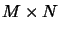: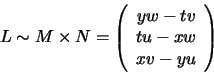Since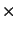is bilinear, the mapping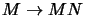for fixed Nis a linear mapping defined by the matrix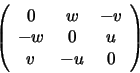The vector N generates the kernel of this mapping.

Another way to characterize the line MN is as the set of points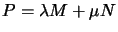for arbitrary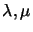. Evidently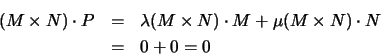Dually, if L and L' are two lines defined by their dual coordinates, then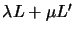is some other line through the intersection X of L and L' (since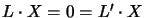implies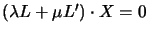). As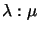varies, this line traces out the entire pencil of lines through X. By duality,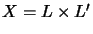.

One further way to approach lines is to recall that if M, N and Pare collinear, each point is a linear combination of the two others. In particular, the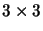determinant |MNP| vanishes. If M and Nare fixed, this provides us with a linear constraint that P must satisfy if it is to lie on the line: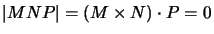.Next: The Fixed Points of Up: Linear Algebra and Homogeneous Previous: Linear Algebra and Homogeneous
Bill Triggs
1998-11-13